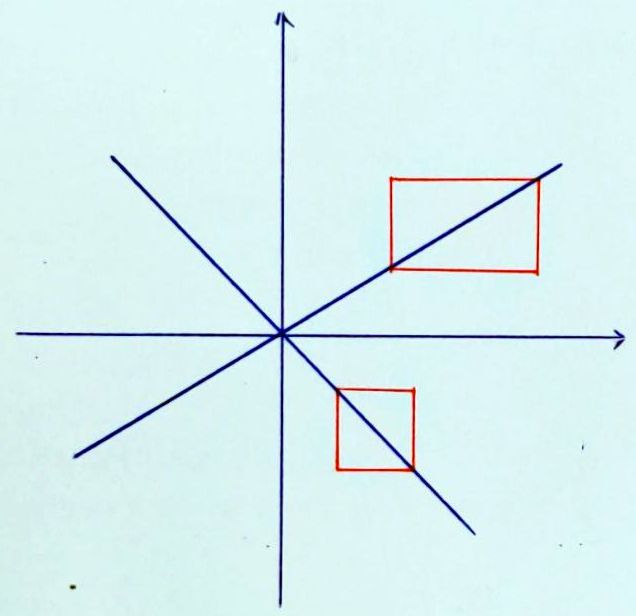# 直線方程式

$$x_0$$ 可讀作 x sub zero 或 x zero，但是美國口語常說 x nought。 直線 line 的形容詞是 linear，而 linear 譯為「線性」或「線型」。

「數學是發現還是發明？」：

Is Mathematics Invented or Discovered?

$$x$$ 坐標是水平的，$$y$$ 坐標是鉛直的。
The $$x$$ axis is horizontal and the $$y$$ axis is vertical.參閱右圖， 在一條既不水平也不鉛直的直線上任取兩點，可以決定一個由水平、鉛直邊組成的長方形， 使得直線是長方形的對角線。 當直線是通過長方形左下角的對角線，我們說長：寬的比值是直線的斜率 (slope 或 gradient)； 當直線是通過長方形左上角的對角線，則規定長寬比的相反數是直線的斜率。

The slope of a horizontal line is 0, and the vertical lines have no slopes.

$$x$$ 截距和 $$y$$ 截距分別是 $$x$$-intercept 和 $$y$$-intercept。 直線與 $$x$$ 軸正向 (the positive direction of the $$x$$ axis) 所夾的有向角 (directed angle) 稱為傾角 (angle of inclination)， 斜率等於傾角的正切：

The slope equals the tangent of the angle of inclination.

 [語音講解：line-eq.mp3]

[ 回上層 ]

Created: Sep 5, 2005
Last Revised: 2022/02/28, 08/20, 12/07